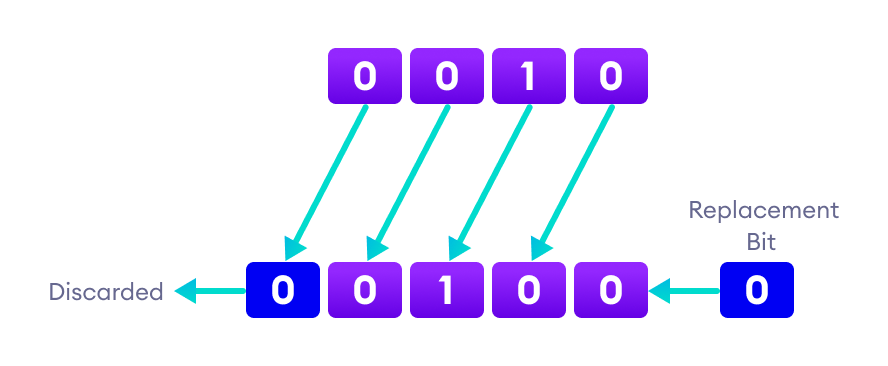# java入门教程 1.3 - 运算符

## Java tutorial 1.3 operator

Posted by xuepro on December 17, 2022

## 1.3 运算符、表达式、语句、程序块 （operator, Expressions, Statements, and Blocks）

### 1.3.1 运算符（operator）

算术运算符



1. 算术运算符

int x = 5 % 3;            // x = 2
double y = .5 % .3;       // y = .2


2.关系运算符

int a = 3, b = 5;
System.out.println(a < b);  // true
System.out.println(a > b);  // false
System.out.println(a >= b);  // false
System.out.println(a <= b);  // true
System.out.println(a == b);  // false
System.out.println(a != b);  // true


instanceof用于测试一个对象是否是指定类型（类或子类或接口）的实例。今后会介绍这个运算符。

3. 逻辑运算符

运算符 例子 含义
&& exp1 && exp2 仅当 exp1 和 expr2都为true时才为true
|| exp1 || exp2 如果 exp1 或 exp2有一个为true，则为true
!exp 如果exp为false则为true，如果exp为true则为false

class TestLogicalOperators{
public static void main(String[] args) {
int a = 7;
int b = 2；
int c = 5;

System.out.println((a > b) && (b > c));  // false
System.out.println((a > b) || (b > c));  // true
System.out.println(!(a == b));  // true
System.out.println(!(a > b));  // false
}
}


4.按位运算符

class TestBitwiseComplement {
public static void main(String[] args) {
int x = 23;
System.out.println(x);                                 //输出23
System.out.println(Integer.toBinaryString(x));         //输出10111

int y  = ~x;
System.out.println(y);                                 //输出-24
System.out.println(Integer.toBinaryString(y));         //输出11111111111111111111111111101000
}
}


 **按位或 **：

class TestBitwiseOR {
public static void main(String[] args) {
int x = 45;
System.out.println(Integer.toBinaryString(x));
int y = 27;
System.out.println(Integer.toBinaryString(y));
int z  = x|y;
System.out.println(Integer.toBinaryString(z));
System.out.println(z);
}
}


45&27的结果是9（其二进制的最后4位是1001，其余位是0）。

45^27的结果是54（其二进制的最后6位是110110，其余位是0）。例如，45«2的结果是180（对应二进制位10110100）。

class TestSignedRightShiftTest{
public static void main(String[] args) {
int x = -4;
System.out.println(Integer.toBinaryString(x));         // 输出：11111111111111111111111111111100
System.out.println(Integer.toBinaryString(x>>1));      // 输出：11111111111111111111111111111110
System.out.println(x>>1);                              // 输出：-2

int y = 4;
System.out.println();
System.out.println(Integer.toBinaryString(y));         // 输出：100
System.out.println(Integer.toBinaryString(y>>1));      // 输出：10
System.out.println(y>>1);                              // 输出：2
}
}


 class TestUnsignedRightShiftTest{
public static void main(String[] args) {
int x = -4;
System.out.println(Integer.toBinaryString(x));         // 输出：11111111111111111111111111111100
System.out.println(Integer.toBinaryString(x>>1));      // 输出：11111111111111111111111111111110
System.out.println(x>>1);                              // 输出：-2

int y = 4;
System.out.println();
System.out.println(Integer.toBinaryString(y));         // 输出：100
System.out.println(Integer.toBinaryString(y>>1));      // 输出：10
System.out.println(y>>1);                              // 输出：2

System.out.println();
System.out.println(Integer.toBinaryString(x>>>1));     // 输出：1111111111111111111111111111110
System.out.println(x>>>1);                             // 输出：2147483646
System.out.println(Integer.toBinaryString(y>>>1));     // 输出：10
System.out.println(y>>>1);                             // 输出：2
}
}


5. 赋值运算符

int age;
age = 15;


“age = 15”将整数15赋值给int类型变量age。赋值运算符可以和算术运算符或位运算符结合，构成复合赋值运算符。如：= += -= *= /= %= &= ^= |= <<= >>= >>>=。 对于变量a和运算数b，“a+=b”相当于“a = a+b”。其余的复合赋值运算符以此类推。

6.一元运算符 一元运算符仅对一个操作数进行运算。例如，++ 是一个一元运算符，它将变量的值增加 1。也就是说，++2 将返回 3。如下表所示，共有5个一元运算符。 |运算符|含义| 变量 | 运算 | 输出值 | | - | — | – | – | – | | + | 一元加号：没有必要使用，因为数字默认就是正数 | int a=3 | +a | 3| | - | 一元负号：反转运算数的符号 | int a=3 | -a | -3 | | ++ | 自增运算符：将值递增 1 |int a=3 | ++a | 4 | | - | 自减运算符：将值减 1 |int a=3 | –a | 2 | | ！ | 逻辑补码运算符：反转布尔值 |boolean a=true | !a | false |

++放在运算符的前面，称为前缀++，如++a。也可以放在运算符的后面，称为后缀++。如a++。

a = 2
++a;          // a becomes 3
a++;          // a becomes 4
--a;          // a becomes 3
a--;          // a becomes 2


• 如果使用 ++ 运算符作为前缀，如：++a，则 a 的值递增 1；然后返回这个递增后的a的值。
• 如果使用 ++ 运算符作为后缀，如：a++，则首先返回a的原始值；然后 a 增加 1。
class TestPrefixPostfix {
public static void main(String[] args) {
int a = 2;
int  b =++a;
System.out.println(a);        // 输出: 3
System.out.println(b);        // 输出: 3
a=2;
b = a++;
System.out.println(a);        // 输出: 3
System.out.println(b);       // 输出: 2
}
}


7.条件运算符 ?: 三元运算符（条件运算符）?: 是 条件语句if-then-else （条件语句将在语句一节内介绍）的简写。格式为： Expression ? expression1 : expression2 其含义是：当Expression为true或非零值时，该表达式的结果为expression1，否则，结果为expression2。

float score = 70.5f;
string result = score>=60? "you passed!":"you failed!";
System.out.println(result);               // 输出： you passed!